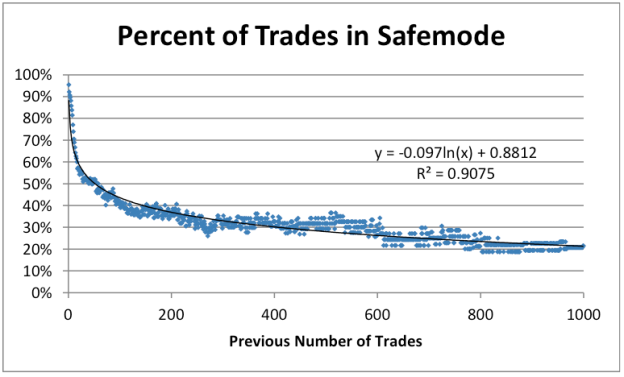# Revision: Analysis of SciCast Safe Mode Usage

The post Analysis of SciCast Safe Mode Usage has been revised slightly to use a power law (x^k) instead of a logarithmic function (k ln x). This is because the logarithmic function could go negative, which the percentage of trades cannot do. Revisions are in red.

0# Analysis of SciCast Safe Mode Usage

by Kellen Leister

Note: The following post has been revised slightly to use a power law (x^k) instead of a logarithmic function (k ln x). This is because the logarithmic function could go negative, which we know the percentage of trades cannot do. Revisions are in red.

Given the ease of switching back and forth between Safe Mode and Power Mode, we were interested to observe the relative use of each mode.  (For a description of the different modes, see the Appendix.) Continue reading

2+